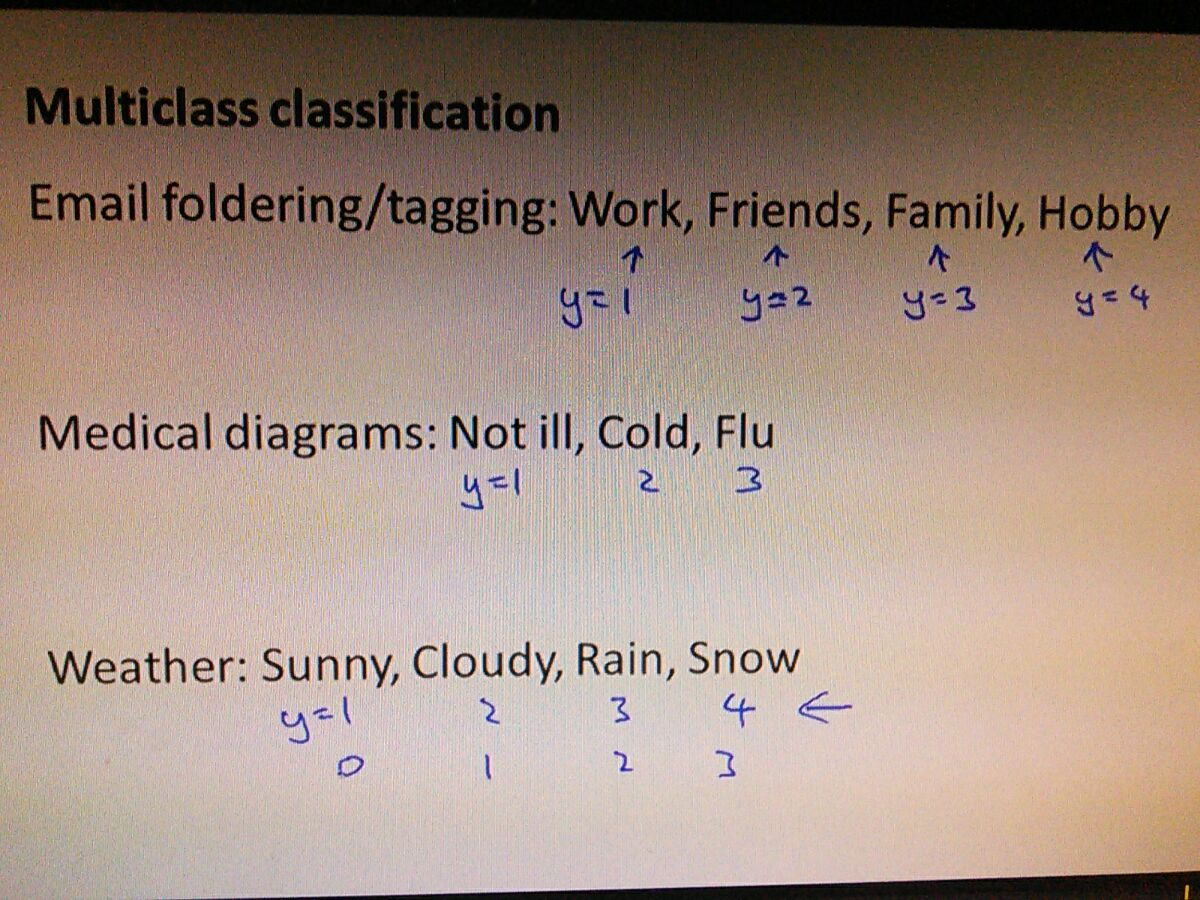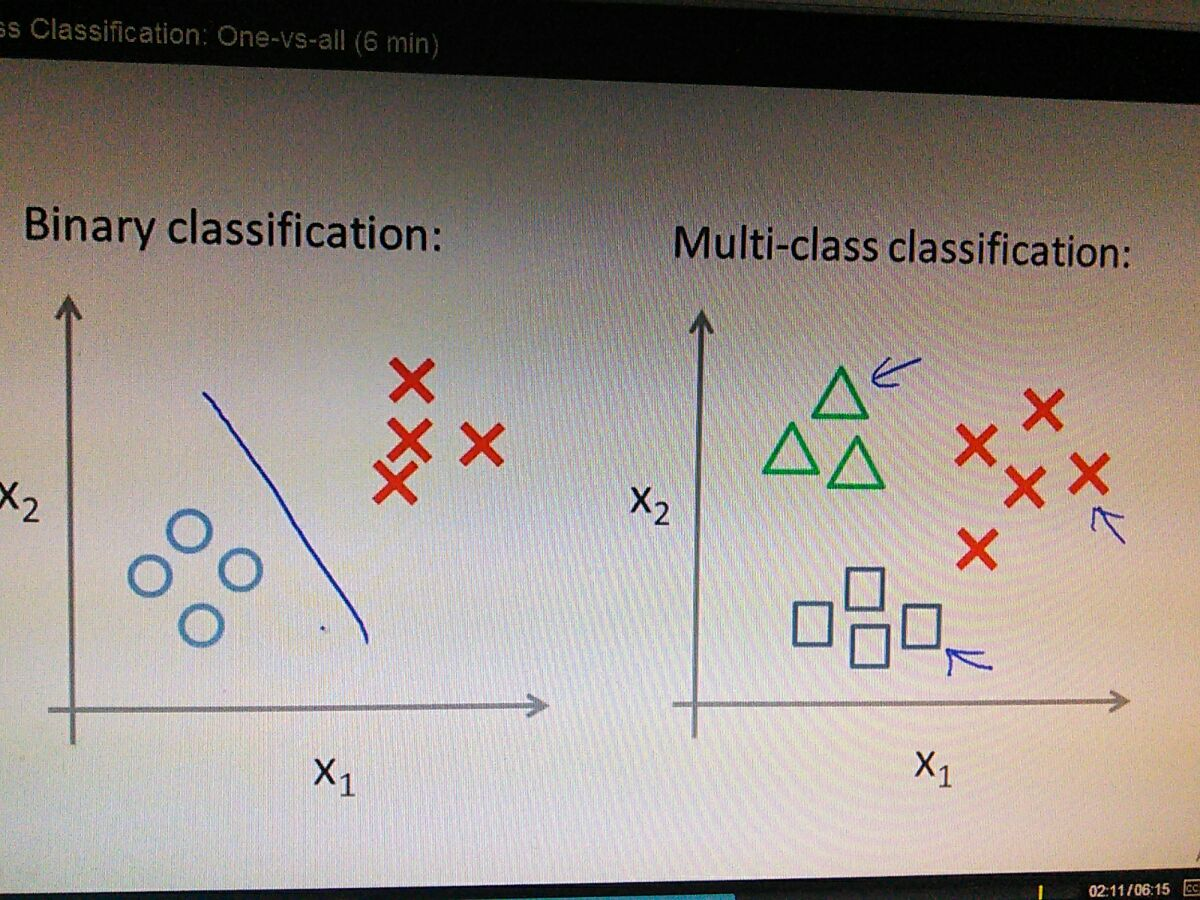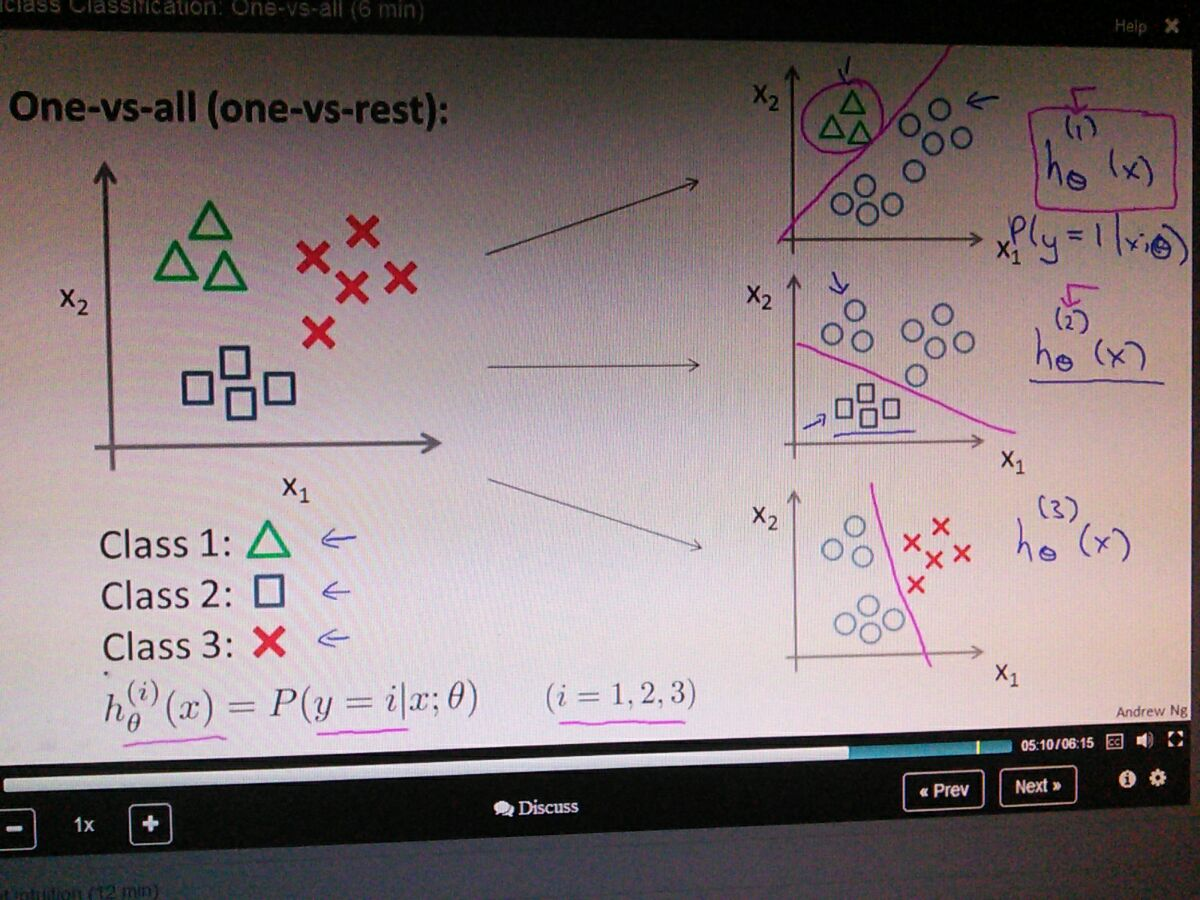-the one that called one-vs-all classification algorithm
classification with more than two groupseither starting from index 0 or 1 doesn't matter..the defined algorithms earlier is to compute the binary by using logistic regression...
using one vs all algorithm for solving multiclass classification.• the algorithm is essentially make two groups,  with each class again the rest...and take each hypothesis(that denotes by superscript) for each class